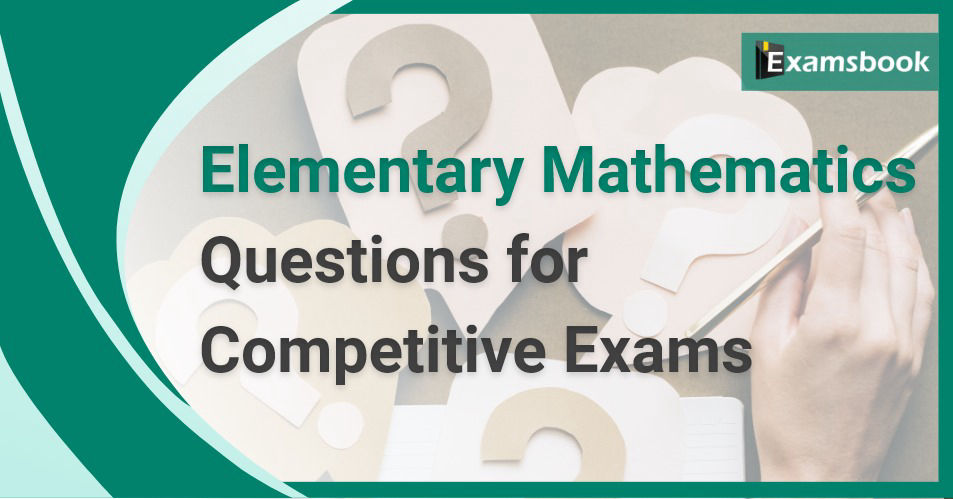• Save

# Elementary Mathematics Questions and Answers

Vikram Singh6 months ago 2.7K ViewsIf you are interested in mathematics, then elementary mathematics can prove to be a very easy subject for you. The topics coming in this subject are easy. If you practice more and more of these topics, then you can get maximum marks not only in Mathematics subject but also in competitive examination.

Here I am giving some very important questions related to the primary subject, practice these questions as much as possible and get maximum marks in the competitive examination.

## Elementary Mathematics Questions

Q :

The average of the marks obtained in an examination by 8 students was 51 and by 9 other students was 68. The average marks of all 17 students was:

(A) 59

(B) 59.5

(C) 60

(D) 60.5

Correct Answer : C

Q :

A library has an average number of 510 visitors on Sunday and 240 on other days. The average number of visitors per day in a month of 30 days beginning with Sunday is:

(A) 300

(B) 290

(C) 285

(D) 295

Correct Answer : C

Q :

12 kg of rice costing 30 per kg is mixed with 8 kg of rice costing 40 per kg. The average per kg price of mixed rice is

(A) 35

(B) 34

(C) 38

(D) 37

Correct Answer : B

Q :

A can do a piece of work in 10 days and B can do it in 15 days. They work together for 5 days, the rest of the work is finished by C in two more days. If they got ₹ 6000 as wages for the whole work, then find the daily wages of A, B and C.

(A) 600, 400, 500

(B) 400,600 , 800

(C) 400, 500, 600

(D) None of these

Correct Answer : A

Q :

A goldsmith observes that one of his employees prepares a necklace in 9 hours, while another employee prepares it in 10 hours. If they work together, they stud 10 gems less every hour and thus prepare a necklace in 5 hours, then the number of gems studded in each necklace is:

(A) 1200

(B) 1000

(C) 800

(D) 900

Correct Answer : D

Q :

P and Q can do a piece of work in 12 days, Q and R can do a piece of work in 15 days and R and P can do a piece of work in 20 days. Q alone can do the whole work in

(A) 25 days

(B) 30 days

(C) 20 days

(D) 24 days

Correct Answer : C

Q :  If A:B:C=2:3:4, then the ratio$$({a\over b}):({b\over c}):({c\over a})$$will be

(A) 8:9:16

(B) 8:9:12

(C) 8:9:24

(D) 4:9:16

Correct Answer : C

Q :

If the compound ratio of [(3a + 2b) : 3] and [1 : (3a + b)] is 1 : 2, find the ratio a : b.

(A) 1 : 4

(B) 1 : 6

(C) 1 : 2

(D) 1 : 3

Correct Answer : D

Q :

Find the mean proportional between 8 and 98.

(A) 112

(B) 28

(C) 16

(D) 53

Correct Answer : C

Q :

The cost price of a book is ₹110 and the selling price is ₹123.20. What percent profit will the bookseller make on selling it?

(A) 11%

(B) 12%

(C) 13%

(D) 14%

Correct Answer : B

### Vikram Singh

Providing knowledgable questions of Reasoning and Aptitude for the competitive exams.